# 机械系统的频率响应分析

2019年 6月 5日

### 什么是频率响应？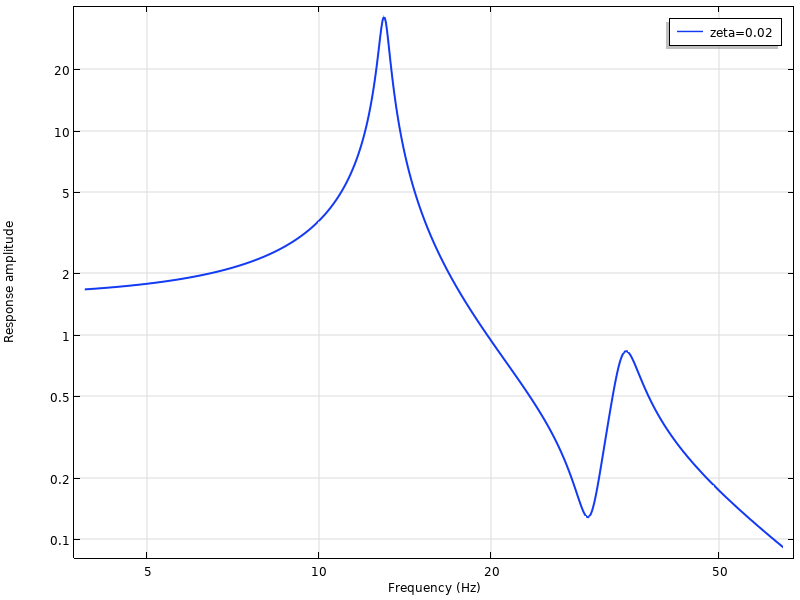### ﻿重新审视单自由度系统﻿

\omega_d = \omega_0\sqrt{1-\zeta^2} \approx \omega_0 \left ( 1 – \frac{\zeta^2}{2} \right )\left (-\omega^2m +ic\omega +k \right) u = f

\left (1-\left (\frac{\omega}{\omega_0} \right) ^2 +2i\zeta \left (\frac{\omega}{\omega_0} \right) \right) u = \frac{f}{k}

\displaystyle H(\omega) = \left (1-\left (\frac{\omega}{\omega_0} \right) ^2 +2i\zeta \left (\frac{\omega}{\omega_0} \right) \right)^{-1} =\frac{1}{1-\beta ^2 +2i\zeta \beta}

H 函数通常称作传递函数。其中 β 表示激励频率与无阻尼固有频率的比值。传递函数的大小为。

\displaystyle \left | \frac{1}{1-\beta ^2 +2i\zeta \beta} \right | = \frac{1}{\sqrt {(1-\beta ^2)^2 +4\zeta^2 \beta^2}}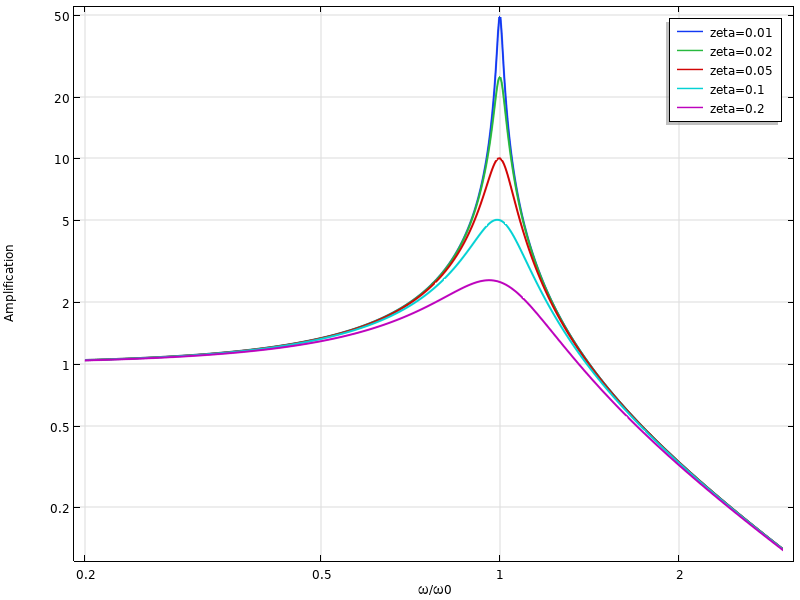\beta = \sqrt{1-2 \zeta^2}

\omega_{\mathrm {max}} = \omega_0\sqrt{1-2\zeta^2} \approx \omega_0 \left ( 1 – \zeta^2 \right )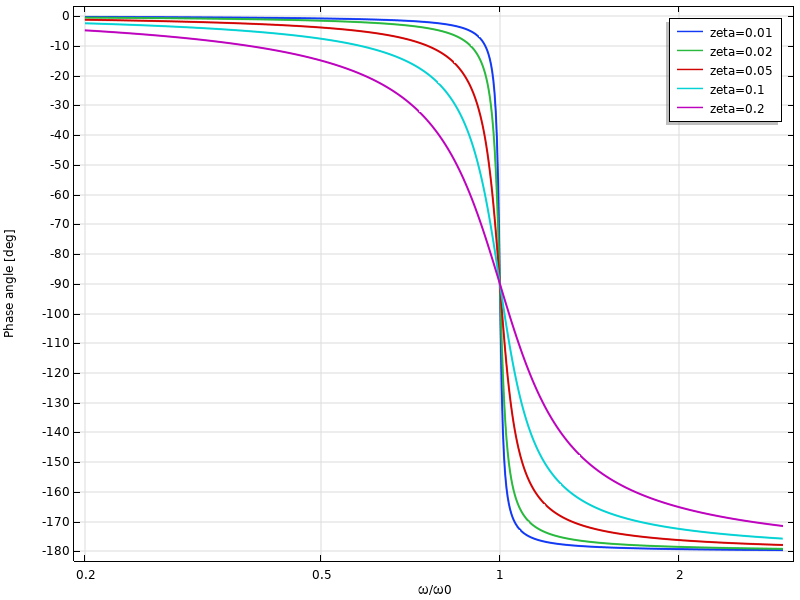### 损耗因子的阻尼

\left (-\omega^2m +k(1+i\eta ) \right) u = f

\displaystyle \omega_d = \omega_0 \sqrt {\left( \frac{1}{2}
\left( 1 + \sqrt{1+\eta^2} \right ) \right ) } \approx \omega_0 \left (1 + \frac{\eta^2}
{8} \right )

|\tilde k| = k \sqrt {1 + \eta^2} \approx k \left ( 1+ \frac{\eta^2}{2} \right )

\displaystyle \frac{1}{1-\beta ^2 +i\eta }

\displaystyle \left | \frac{1}{1-\beta ^2 +i\eta} \right | = \frac{1}{\sqrt {(1-\beta ^2)^2 +\eta^2}}

\tilde k = \displaystyle \frac{k(1+i \eta)}{\sqrt{1+ \eta^2}}

\displaystyle \omega_d = \omega_0 \sqrt { \frac {\frac{1}{2} \left( 1 + \sqrt{1+\eta^2} \right )}{1+ \eta^2} } \approx \omega_0 \left (1 – \frac{3\eta^2}{8}
\right )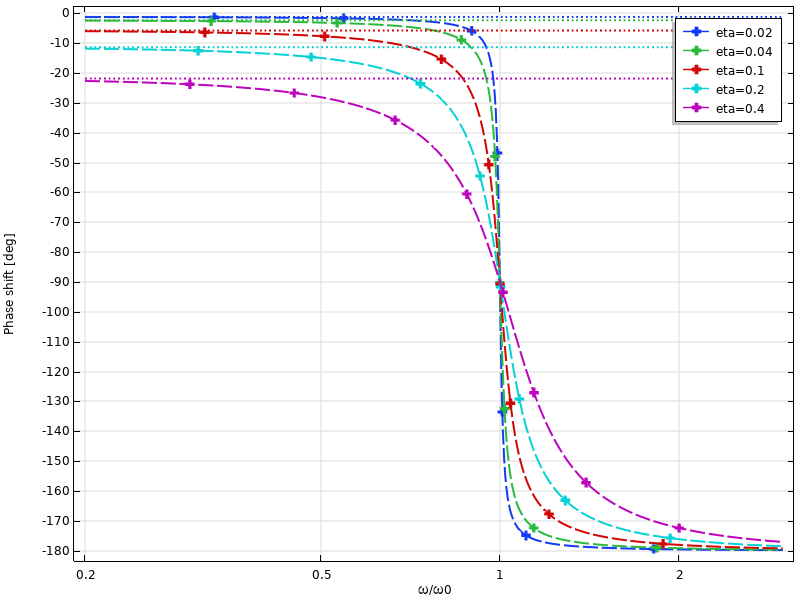### 在 COMSOL Multiphysics® 中进行频率响应建模

#### 建立研究

1. 频域
2. 频域，预应力
3. 频域，模态
4. 频域，预应力，模态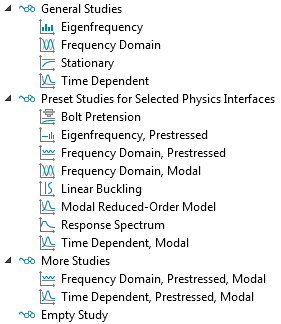Solid Mechanics 界面的可用学习类型。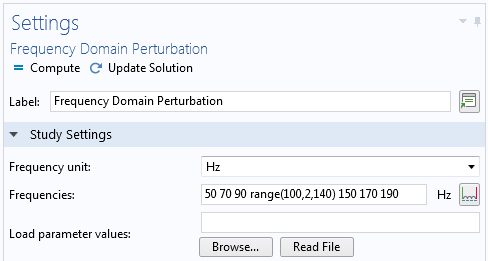#### 是否存在扰动？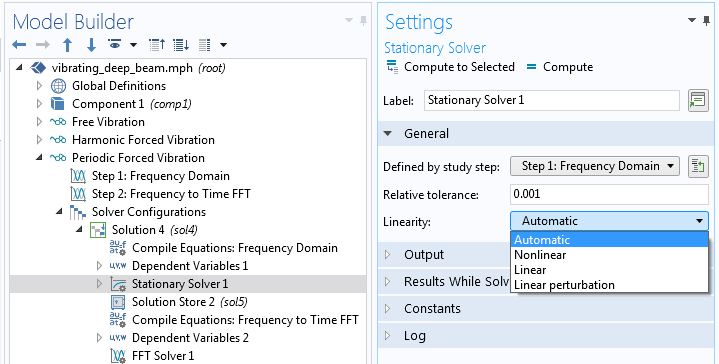#### 解释结果

\displaystyle v = \Re(\tilde v e^{i \phi})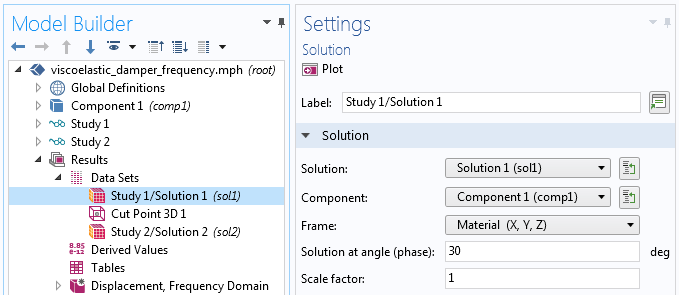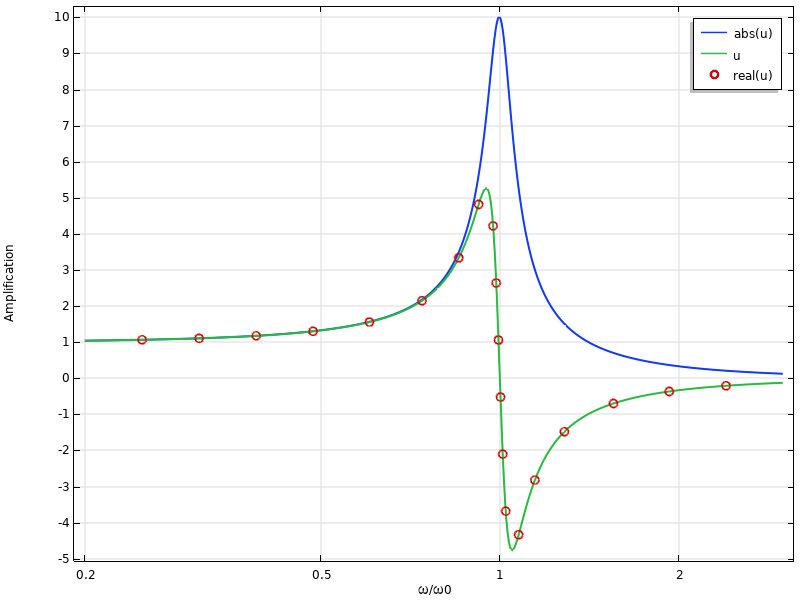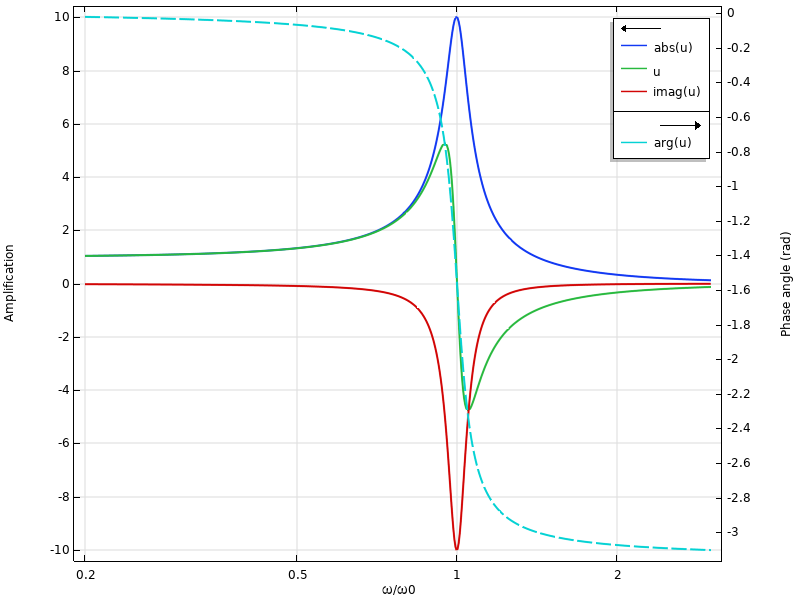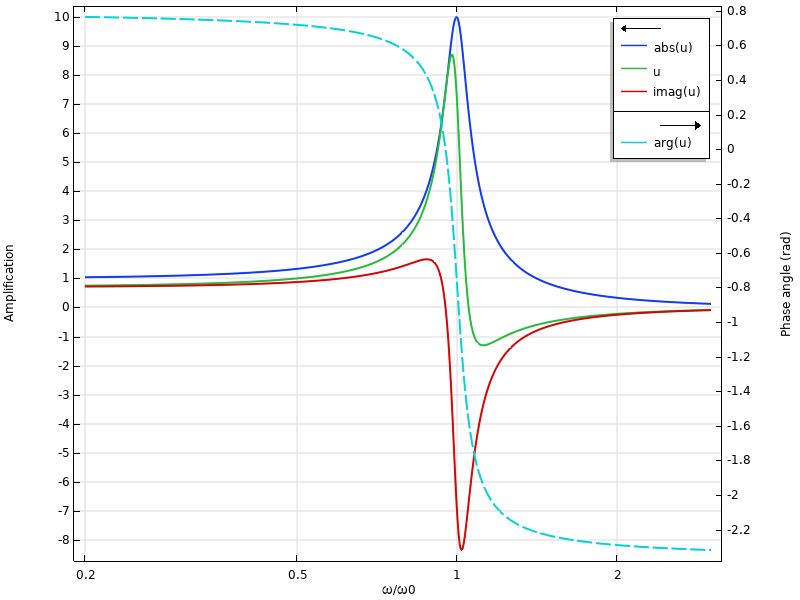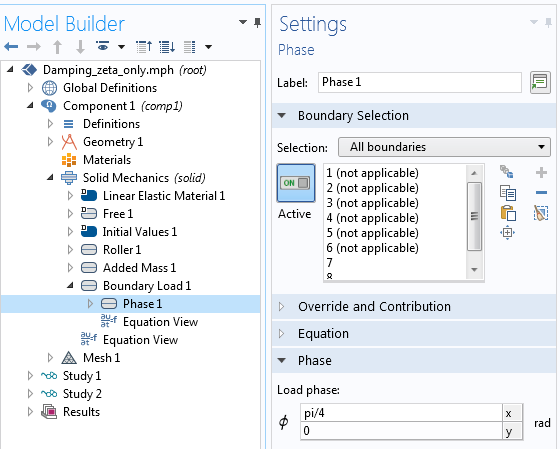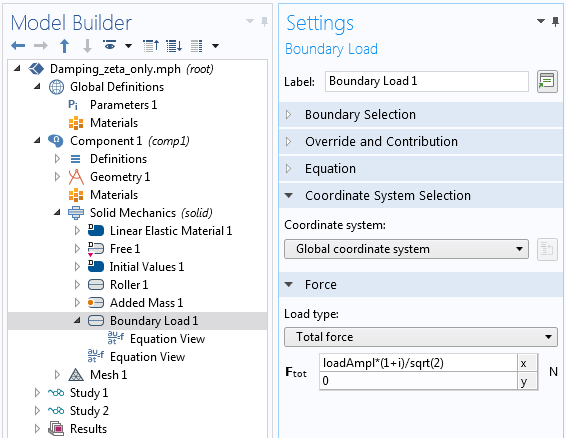##### 扰动研究的结果### 将频率响应结果转换为时域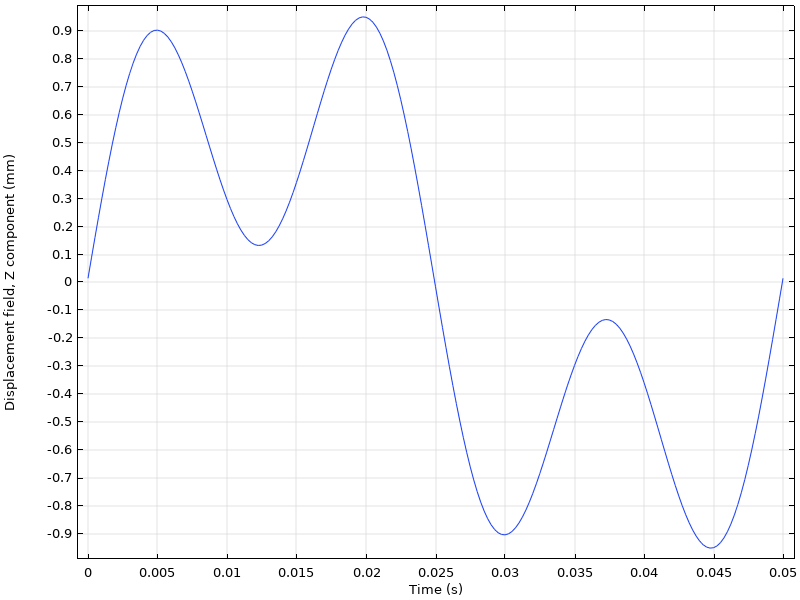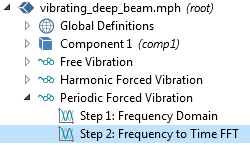### 结束语

COMSOL 案例库中还有更多机械频率响应分析示例，例如：

#### 评论 (1)

##### 留言2019-10-22